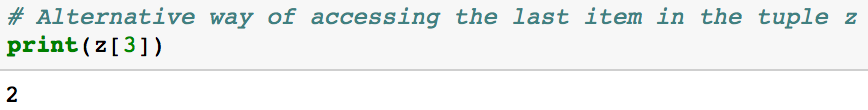# Python Tuples and Tuple Methods

June 22nd 2017

Tuples are an ordered sequences of items, just like lists. The main difference between tuples and lists is that tuples cannot be changed (immutable) unlike lists which can (mutable).

### Initialize a Tuple

There are two ways to initialize an empty tuple. You can initialize an empty tuple by having () with no values in them.

``# Way 1emptyTuple = ()``

You can also initialize an empty tuple by using the `tuple` function.

``# Way 2emptyTuple = tuple()``

A tuple with values can be initialized by making a sequence of values separated by commas.

``# way 1z = (3, 7, 4, 2)``
`# way 2 (tuples can also can be created without parenthesis)z = `3, 7, 4, 2``

It is important to keep in mind that if you want to create a tuple containing only one value, you need a trailing comma after your item.

`# tuple with one valuetup1 = ('Michael',)`
`# tuple with one valuetup2 = 'Michael', `
`# This is a string, NOT a tuple.notTuple = ('Michael')`

### Accessing Values in Tuples

Each value in a tuple has an assigned index value. It is important to note that python is a zero indexed based language. All this means is that the first value in the tuple is at index 0.

`# Initialize a tuplez = (3, 7, 4, 2)`
`# Access the first item of a tuple at index 0print(z)`

Python also supports negative indexing. Negative indexing starts from the end of the tuple. It can sometimes be more convenient to use negative indexing to get the last item in a tuple because you don’t have to know the length of a tuple to access the last item.

`# print last item in the tupleprint(z[-1])`

As a reminder, you could also access the same item using positive indexes (as seen below).Alternative way of accessing the last item in the tuple z

### Tuple slices

Slice operations return a new tuple containing the requested items. Slices are good for getting a subset of values in your tuple. For the example code below, it will return a tuple with the items from index 0 up to and not including index 2.First index is inclusive (before the :) and last (after the :) is not
`# Initialize a tuplez = (3, 7, 4, 2)`
`# first index is inclusive (before the :) and last (after the :) is not.print(z[0:2])`
`# everything up to but not including index 3print(z[:3])`

You can even make slices with negative indexes.

`print(z[-4:-1])`

### Tuples are Immutable

Tuples are immutable which means that after initializing a tuple, it is impossible to update individual items in a tuple. As you can see in the code below, you cannot update or change the values of tuple items (this is different from Python Lists which are mutable).

`z = (3, 7, 4, 2)`
`z = "fish"`

Even though tuples are immutable, it is possible to take portions of existing tuples to create new tuples as the following example demonstrates.

`# Initialize tupletup1 = ('Python', 'SQL')`
`# Initialize another Tupletup2 = ('R',)`
`# Create new tuple based on existing tuplesnew_tuple = tup1 + tup2;print(new_tuple)`

### Tuple Methods

Before starting this section, let’s first initialize a tuple.

`# Initialize a tupleanimals = ('lama', 'sheep', 'lama', 48)`

#### index method

The index method returns the first index at which a value occurs.

`print(animals.index('lama'))`

#### count method

The count method returns the number of times a value occurs in a tuple.

`print(animals.count('lama'))`

### Iterate through a Tuple

You can iterate through the items of a tuple by using a for loop.

`for item in ('lama', 'sheep', 'lama', 48):   print(item)`

### Tuple Unpacking

Tuples are useful for sequence unpacking.

`x, y = (7, 10);print("Value of x is {}, the value of y is {}.".format(x, y))`

### Enumerate

The `enumerate` function returns a tuple containing a count for every iteration (from start which defaults to 0) and the values obtained from iterating over a sequence:

`friends = ('Steve', 'Rachel', 'Michael', 'Monica')for index, friend in enumerate(friends):    print(index,friend)`

### Advantages of Tuples over Lists

Lists and tuples are standard Python data types that store values in a sequence. A`tuple` is immutable whereas a `list` is mutable. Here are some other advantages of tuples over lists (partially from Stack Overflow)

• Tuples are faster than lists. If you’re defining a constant set of values and all you’re ever going to do with it is iterate through it, use a tuple instead of a list. The performance difference can be partially measured using the `timeit` library which allows you to time your Python code. The code below runs the code for each approach 1 million times and outputs the overall time it took in seconds.
`import timeit `
`print(timeit.timeit('x=(1,2,3,4,5,6,7,8,9,10,11,12)', number=1000000))`
``print(timeit.timeit('x=[1,2,3,4,5,6,7,8,9,10,11,12]', number=1000000))``
• Some tuples can be used as dictionary keys (specifically, tuples that contain immutable values like strings, numbers, and other tuples). Lists can never be used as dictionary keys, because lists are not immutable (you can learn about dictionaries here).
• Tuples can be used as values in sets whereas lists can not (you can learn more about sets here)

### Conclusion

If you have any questions or thoughts on the tutorial, feel free to reach out in the comments below or through Twitter. Next post reviews Python Dictionaries and Dictionary Methods.